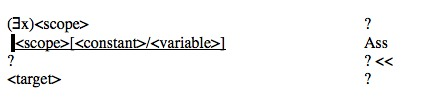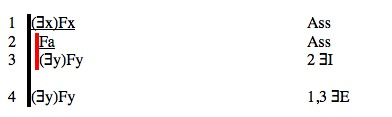# Tutorial 21: Existential Elimination

Logical System

2013

Bergmann The Logic Book Section 10.1.

### The Tutorial

Existential Elimination (often called 'Existential Instantiation') permits you to remove an existential quantifier from a formula which has an existential quantifier as its main connective. It is one of those rules which involves the adoption and dropping of an extra assumption (like ∼I,⊃I,∨E, and ≡I).

The circumstance that Existential Instantiation gets invoked looks like this.

 (∃x) ? ? ? << ?

That is, a formula you have has an existential quantifier as its main connective-- and you are trying to obtain some formula which we will call <target>.

For example,

 (∃x)(Fx) ? ? ? << (∃y)(Fy) ?

invites the use of EE. In this case (∃x)(Fx) is the existential formula, (Fx) is its scope, and (∃y)(Fy) is the target.

To use EE you follow a three stage process. First you assume the <scope>[<constant>/<variable>]. The illustrated fragment will then becomeSecond you continue deriving in this new context until you manage to derive <target> thusFinally you select <target> at the end of the sub-proof, select the existential formula, and click ∃E to complete the existential instantiation.In the example, the derivation would look likeThere are restrictions on EE. The <constant> must not occur either

• a) in the assumptions of the final <target> line,
• or b) in the existential formula that ∃E is used on,
• or c) in the <target>.

Notice that the constant 'a' does not occur in the assumptions of line 4 (which are (∃x)Fx), it does not occur in the existential formula, line 1, and it does not occur in the target, line 4.

More about these restrictions later. The rule is

#### The Rule of Elimination of the Existential Quantifier, Existential Elimination ∃E

If a derivation contains a line of the form

n. <assumptions> (∃<variable>)(<scope>) <any justification>

and a line of the form

k. <assumptions+ <scope[<constant>/<variable>]>> <formula> <any justification>

and

the constant <constant> does not occur in ∃<variable>)(<scope>) , in any of the <assumptions> of line k except the additional assumption <scope[<constant>/<variable>]>, or in the formula <formula>

then a line of the form

<next line number> <assumptions> <formula> 'k EI'

may be added to the derivation.

#### Implementation of EE

You have to have in mind here some existential formula (∃<variable>)<scope> that you are going to instantiate. First use Ass to adopt <scope[<constant>/<variable>]> as a new assumption, You need to pick a 'good' constant here, for it must not occur in all sorts of other places. A good strategy is to pick a brand new constant that does not appear in the derivation at all. Then you derive the <formula> you want as the existential instantiation as the last line of an open sub-proof or sub-derivation. Then select the existential formula (∃<variable>)<scope> and the last line <formula> and press EE. The Rule will check whether <constant> occurs in the existential formula, it will check whether <constant> occurs in the standing assumptions of the intended instantiation <formula>, and it will check whether the <constant> occurs in target <formula>.   The conditions satisfied, the Rule will drop the extra assumption of the sub-derivation and add <formula> as the new line.

## Exercise to accompany Predicate Tutorial 11.

### Exercise 1 (of 3)

It is probably easier to learn EI using Tactics. After you start each derivation, switch Tactics on, select the existentially quantified formula, and click EI.

Derive the following

a) (∃x)(Fa) ∴ Fa
b) (∃x) (Fx&Gx) ∴ (∃x)(Fx )
c) (∃x) (Fx&Gx) ∴ (∃y)(Gy)
d) (∃x)(Fx)∴ (∃y)(Fy)
e) (∃x)(Fx&Gx) ∴ (∃y)(Fy)&(∃z)(Gz)

### Exercise 2 (of 3)

Repeat the derivations of Exercise 1 without using Tactics.

a) (∃x)(Fa) ∴ Fa
b) (∃x) (Fx&Gx) ∴ (∃x)(Fx )
c) (∃x) (Fx&Gx) ∴ (∃y)(Gy)
d) (∃x)(Fx)∴ (∃y)(Fy)
e) (∃x)(Fx&Gx) ∴ (∃y)(Fy)&(∃z)(Gz)

### Exercise 3 (of 3)

Each of following derivations will use Existential Instantiation at some stage.

Derive

a) ∴ (∃x)Fx≡(∃y)Fy
b) (∀x)Fx,(∃x)~Fx∴G
c) ∴ (∃x) (Fx∨Gx) ≡ (∃y)Fy∨(∃z)Gz
d) (∃x)(Fx∨Gx),(∀x)(Fx⊃Hx),(∀x)(Gx⊃Hx)∴(∃x)Hx
e) ~(∃x)(Fx&~Gx),~(∃x)(Gx&~Hx)∴(∀x)(Fx⊃Hx)
f) ~(∃x)Gx,(∀x)(Fx⊃Gx)∴(∀x)(Fx⊃Hx)
g) (∃x)Fx∨(∃x)Gx,(∀x)((Fx∨Gx)⊃Hx)∴(∃x)Hx

If you decide to use the web application for the exercises you can launch it from here Deriver[Bergmann] — username 'logic' password 'logic'. Then either copy and paste the above formulas into the Journal or use the Deriver File Menu to Open Web Page with this address https://softoption.us/test/easyDeriver/CombinedExercisesEasyDBergmann.html .

Preferences

You may need to set some Preferences for this.

• you can check that the parser is set to bergmann.